# [XCS224N] Lecture 2 – Word Vectors and Word Senses

## More on Word2Vec

parameters `θ` : matrix `U` and `V` (each word vec is a row):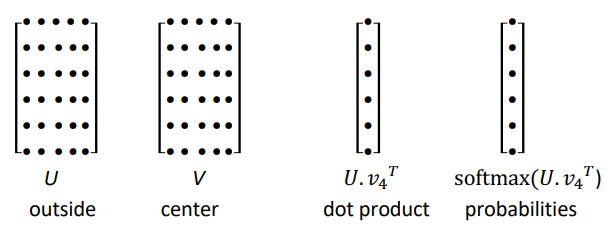and the predictions don't take into account the distance between center word c and outside word o. ⇒ all word vecs predict high for the stopwords.

## Optimization Basics

min loss function: `J(θ)`

direction of the gradient = direction where J(θ) increases the most.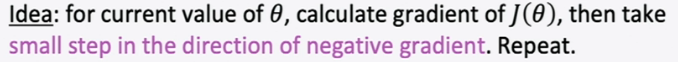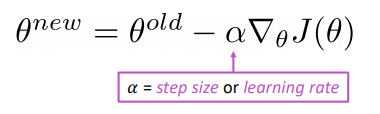#### SGD

pb: `J(θ)` is for all words/positions in the corpus, `grad J(θ)` is very expensive to compute. ⇒ use random samples each time ⇒ each time we only sample one window of `2m+1` words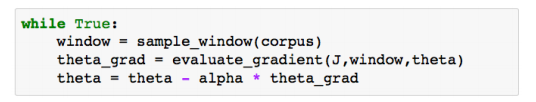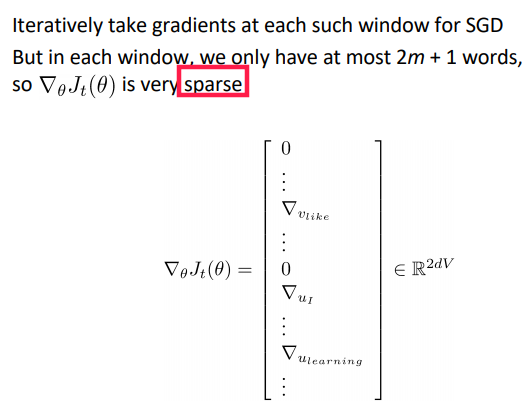I.e.each time we compute the grad only on a minibatch. each time we only update the wordvecs that appear in the minibatch.

## Word2Vec: Model Variants

final embedding of a word = average of `u` and `v` ⇒ we can also do only one vec per word, not much difference

2 main varaints of w2v family:

• skip-grams(SG): predict outside word o with center word c: `P(o|c)` .← presented in the class.
• continuous bag-of-words (CBOW): predict center word c using outside words o.

## negative sampling

So far: naive sotfmax, i.e.sum over all words in vocab — expensive.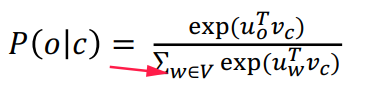negative sampling. Use several binary logistic regressions on:

• the true pair (center word and a true context word )
• several nois pairs (center word and a random word) ← random negative pair

logistic regression = softmax with vocab size=2 sigmoid function: from inner-product value to probability score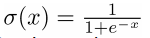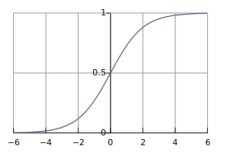⇒ objective funcion (to maximize):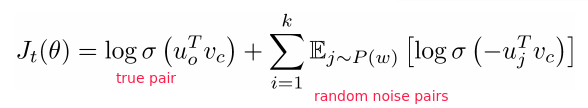where `P(w)` is the distribution of negative sampling. in practice: `P(w)=U(w)^(3/4)` i.e.3/4 power of the uniform distribution → makes rare words appear more often.

⇒ loss function (to minimize):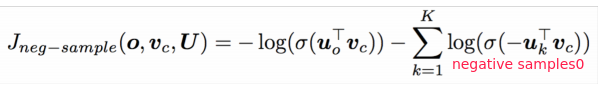QUESTION: why put the minus inside the logit?

## Alternative Methods: cooccurrence counts

Why not using co-occurrence counts matrix?

• co-occurrence in windows
• co-occurrence in document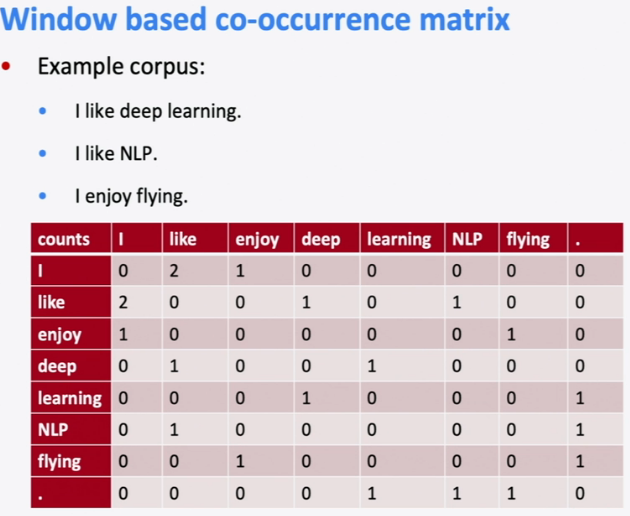problems with co-occurrence matrix:

• matrix size increase with vocab size
• high dimensional, expensive storage
• Subsequent classification models have sparsity issues

model less robust

#### solution1: low-dimensional vectors

(signular value decomposition for k-largest eigen values)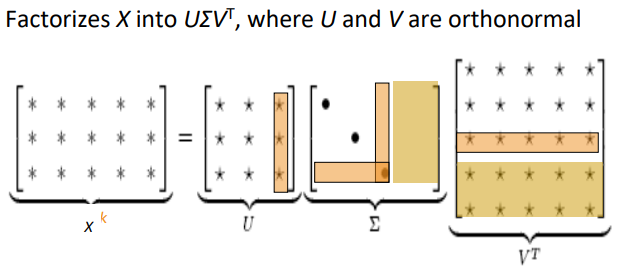⇒ popular in the year 2000.

#### solution2: hacks to co-occurrence matrix

• trim stopwords
• counter more on closer words
• Pearson correlations to replace counts

#### comparison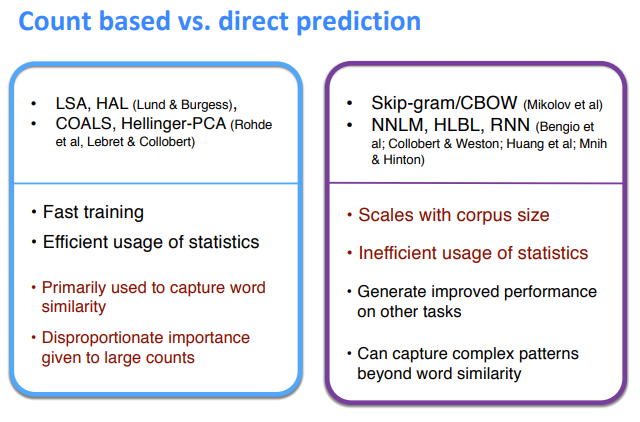#### encode meanings

insight: ratios of co-occurrence probabilities can encode meaning.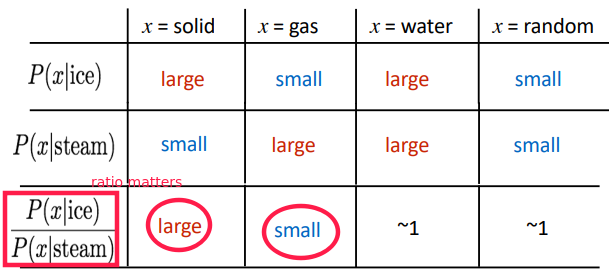make dot-products equal to the log of co-occurence prabability ⇒ vector diff gives the ratio of co-occur proba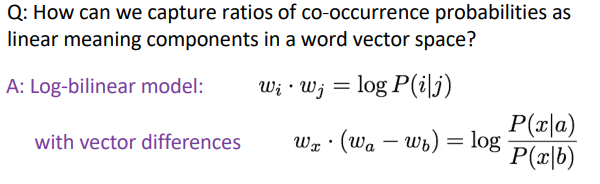## GloVe

Combine the best of both worlds: count method and prediction method.

log-bilinear: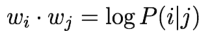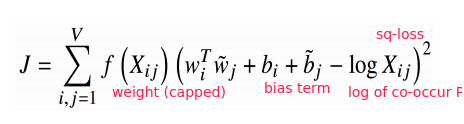Where the weight ter f(Xij) is capped: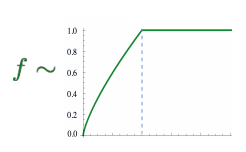• Fast training
• Scalable to huge corpora
• Good performance even with small corpus and small vectors

## Evaluating Word Vectors

Intrinsic vs extrinsic in NLP-eval: Intrinsic:

• Eval on a specific/intermediate subtask: e.g.word similarity, POS tag, etc.
• Fast to compute
• Not clear if really helpful unless correlation to real task is established

Extrinsic:

• Eval on real task: e.g.web search / question-answering / phone dialog
• Hard to run
• Hard to diagnois

⇒ today: focus on intrinsic word vector evaluation

### Eval on word Analogies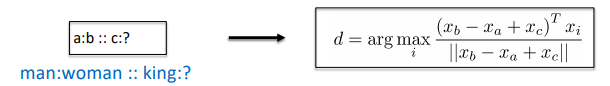• Semantic eval: city-in-state (e.g."Chicago Illinois Houston Texas")
• Syntactic eval: gram4-superlative (e.g."bad worst big biggest")

Hyperparameters: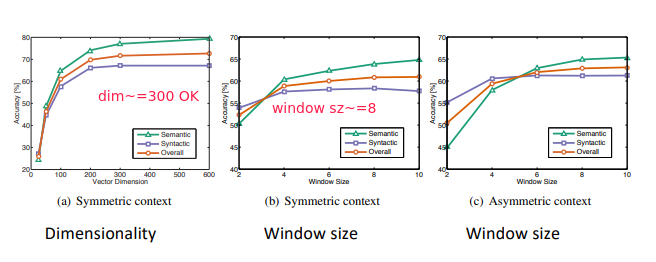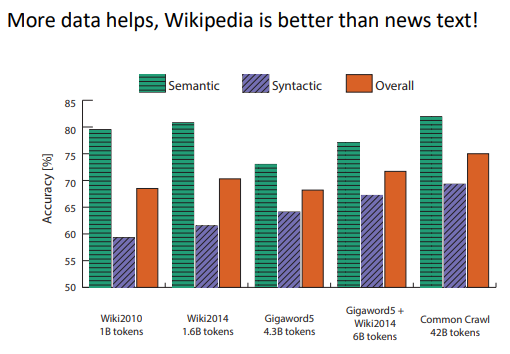### Eval on word Similarities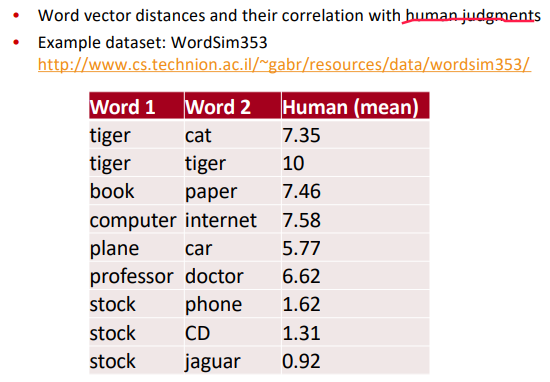## Word Senses

sense ambiguity: words have lots of meanings

• crude solution(2012): for each common word, find clusters of context that it occurs, split the word into pesudowords.
• Linear Algebraic Structure solution (2018):

word sense is a linear superposition: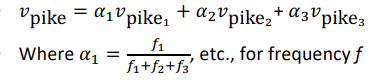⇒ result: Because of ideas from sparse coding you can actually separate out the senses (providing they are relatively common) actual word/sense are very sparse

Extrinsic word vector evaluation: e.g. NER (finding person/organization/location)